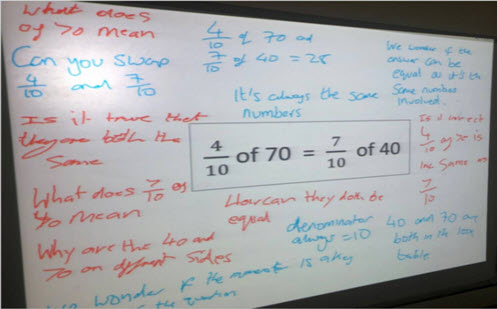Go to website

# Fraction of

Throughout this inquiry students will develop an understanding of how to find a fraction of a quantity where the result is a whole number. Teachers can use the inquiry to explicitly teach the concept and engage students as they discuss the worked example presented. The inquiry provides opportunity for students to deepen their understanding of fractions developing algebraic reasoning.

Year level(s) Year 6
Audience Student, Teacher
Purpose Evidence-based approaches, Extension, Planning support, Teaching resource, Teaching strategies
Teaching strategies and pedagogical approaches Collaborative learning, Differentiated teaching, Explicit teaching, Growth mindset, Mathematics investigation, Metacognitive strategies, Questioning, Worked examples, Structuring lessons
Keywords simple fractions, multiplication, division, numerator, denominator, explicit teaching

## Curriculum alignment

Curriculum connections Numeracy
Strand and focus Number, Apply understanding, Build understanding
Topics Fractions, Operating with number, Decimals, Multiplication and division, Multiples, factors and powers
AC: Mathematics (V9.0) content descriptions
AC9M6N09
Use mathematical modelling to solve practical problems, involving rational numbers and percentages, including in financial contexts; formulate the problems, choosing operations and efficient calculation strategies, and using digital tools where appropriate; interpret and communicate solutions in terms of the situation, justifying the choices made

Multiplicative strategies (P8)
Understanding money (P8)